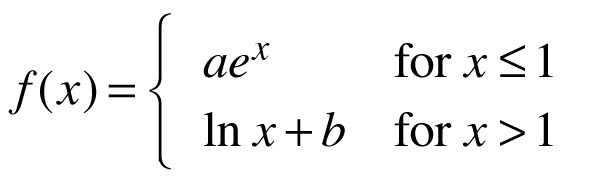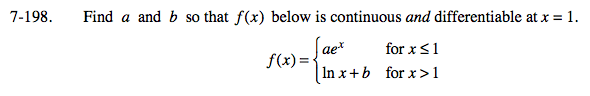### Home > CALC > Chapter Ch7 > Lesson 7.4.3 > Problem7-198

7-198.
1. Find a and b so that f(x) below is continuous and differentiable at x = 1. Homework Help ✎

Homework Help ✎Write and solve a system of equations, each evaluated at x = 1. The first equation will equate the two pieces of f(x).
The second equation will equate the two pieces of f '(x).